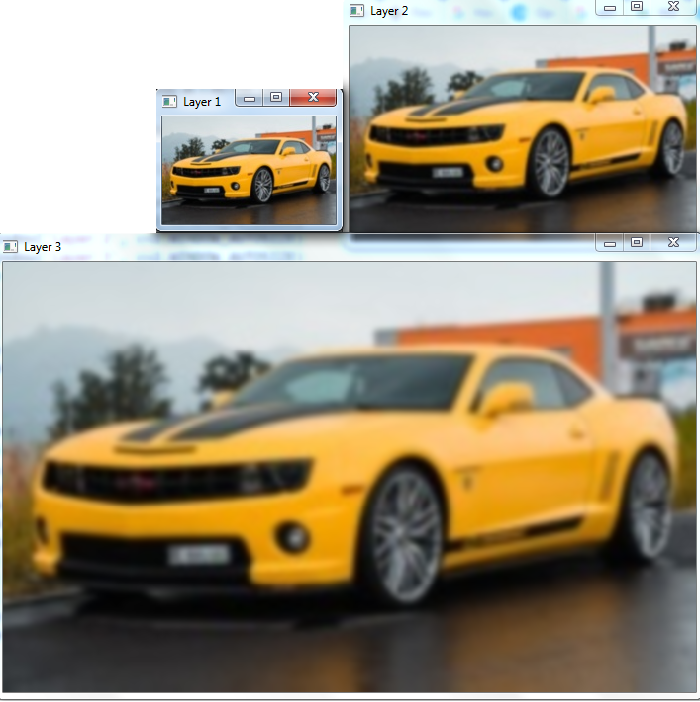# How to find Gaussian pyramids for an image using OpenCV in Python?

In many cases, we need to work with different resolutions and sizes of the same image. In the concept of image pyramid, we find images with different resolutions and sizes of the original image. The Gaussian pyramid is a type of image pyramid.

To find a Gaussian pyramid, OpenCV provides us two functions cv2.pyrDown() and cv2.pyrUp().

• The function cv2.pyrDown() decreases the resolution by removing the consecutive rows and columns in the input image. The width and height of the output image become half the input image decreasing the area to one-fourth.

• The function cv2.pyrUp() increases the resolution by adding the consecutive rows and columns in the input image. The width and height of the output image become double the input image increasing the area to four times.

We can create a Gaussian pyramid by doing level down or level up. To create a level-down Gaussian pyramid, we apply cv2.pyrDown() function. While creating a level-up Gaussian pyramid we apply cv2.pyrUp() function.

In creating the Gaussian pyramids, the original image is set as the base image. The higher levels can be found using these two functions.

## Example 1

In this example, we create a 3-layer Gaussian pyramid. We do the level down two times using cv.pyrDown().

# import the required libraries
import cv2

# Load the image. it is the level 1 of Gaussian Pyramid

# obtain level 2 image (decrease the resolution)
pyramid1 = cv2.pyrDown(img)

# obtain level 3 image of Gaussian Pyramid (decrease the resolution)
pyramid2 = cv2.pyrDown(pyramid1)

# define three windows to display three Level images
cv2.imwrite('pyramid2.jpg', pyramid2)
cv2.namedWindow('Layer 1', cv2.WINDOW_AUTOSIZE)
cv2.namedWindow('Layer 2', cv2.WINDOW_AUTOSIZE)
cv2.namedWindow('Layer 3', cv2.WINDOW_AUTOSIZE)

# Display all the level images
cv2.imshow('Layer 1', img)
cv2.imshow('Layer 2', pyramid1)
cv2.imshow('Layer 3', pyramid2)
cv2.waitKey(0)
cv2.destroyAllWindows()


We will use the following image "car.jpg" as the Input File in the above program code.## Output

On execution, it will produce the following output window.The above output shows that the resolution of the higher level (say Layer 2) is decreased by half of the resolution of the lower level (say Layer 1). In the same way, the resolution of Layer 3 is half of Layer 2.

Note − The output image at Layer 3 obtained above is used as the input to the Example 2 below.

## Example 2

In this example, we create a 3-layer Gaussian pyramid doing level up. We level up two times using cv2.pyrUp().

import cv2

pyr1 = cv2.pyrUp(img)
pyr2 = cv2.pyrUp(pyr1)

cv2.namedWindow('Layer 1', cv2.WINDOW_AUTOSIZE)
cv2.namedWindow('Layer 2', cv2.WINDOW_AUTOSIZE)
cv2.namedWindow('Layer 3', cv2.WINDOW_AUTOSIZE)

cv2.imshow('Layer 1', img)
cv2.imshow('Layer 2', pyr1)
cv2.imshow('Layer 3', pyr2)

cv2.waitKey(0)
cv2.destroyAllWindows()


## Output

On execution of the above code, it will produce the following output window.The above output shows that the resolution of the higher level (say Layer 2) is doubled of the resolution of the lower level (say Layer 1). In the same way the resolution of Layer 3 is double of Layer 2.

Note the difference between the input image in Example 1 and layer 3 image in output of Example 2.

Updated on: 28-Sep-2022

663 Views